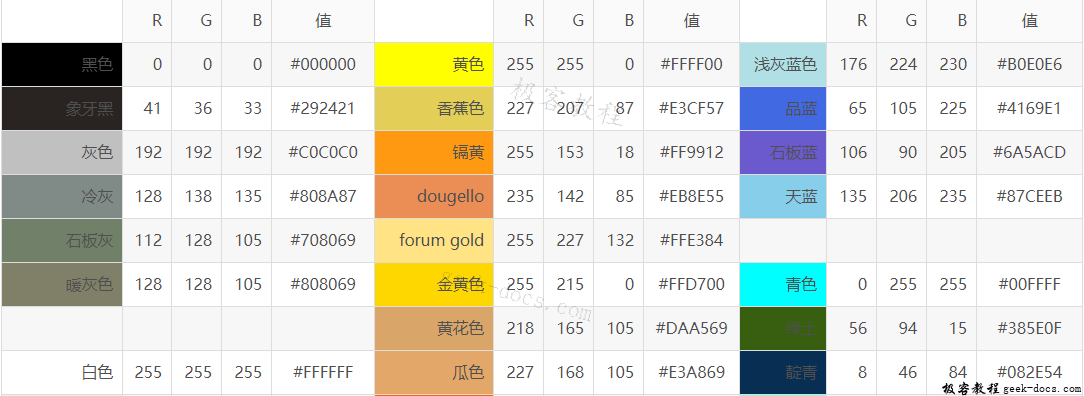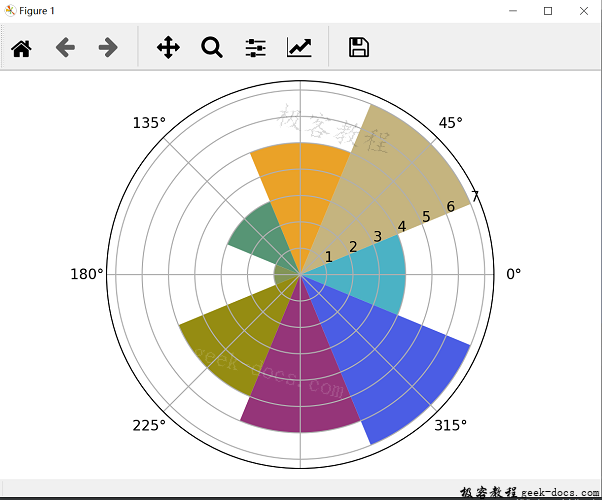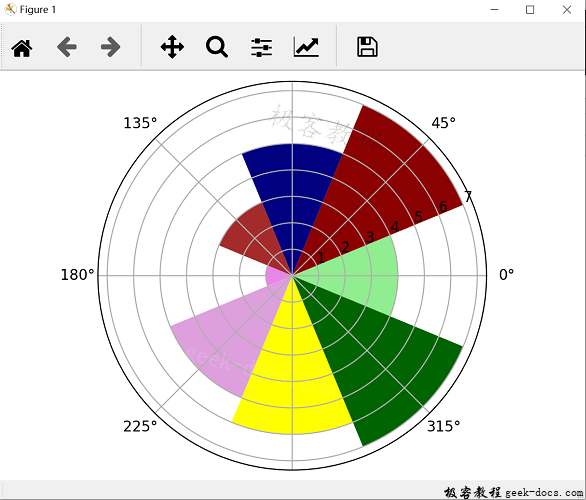# Matplotlib 极区图

Matplotlib 极区图极区图由一系列呈放射状延申的区域组成，其中每块区域占据一定的角度。因此若要用极区图表示两个不同的数值，分别指定它们在极区图中所占的分量：每块区域的半径r和它所占的角度，就是极坐标(r,θ)import matplotlib.pyplot as plt
import numpy as np

N = 8
'''生成角度值'''
theta = np.arange(0.,2*np.pi,2*np.pi/N)
'''生成半径值'''
'''定义轴类型'''
plt.axes([0.025,0.025,0.95,0.95],polar=True)
'''定义颜色集，这里使用RGB值，当然也可以使用颜色名称'''
colors = np.array(['#4bb2c5','#c5b47f','#EAA228','#579575','#839557','#958c12','#953579','#4b5de4'])
'''bar()函数要求传入角度和半径作为参数'''
plt.show()import matplotlib.pyplot as plt
import numpy as np

N = 8
'''生成角度值'''
theta = np.arange(0.,2*np.pi,2*np.pi/N)
'''生成半径值'''
'''定义轴类型'''
plt.axes([0.025,0.025,0.95,0.95],polar=True)
'''定义颜色集，这里使用颜色名称，当然也可以使用RGB值'''
colors = np.array(['lightgreen','darkred','navy','brown','violet','plum','yellow','darkgreen'])
'''bar()函数要求传入角度和半径作为参数'''